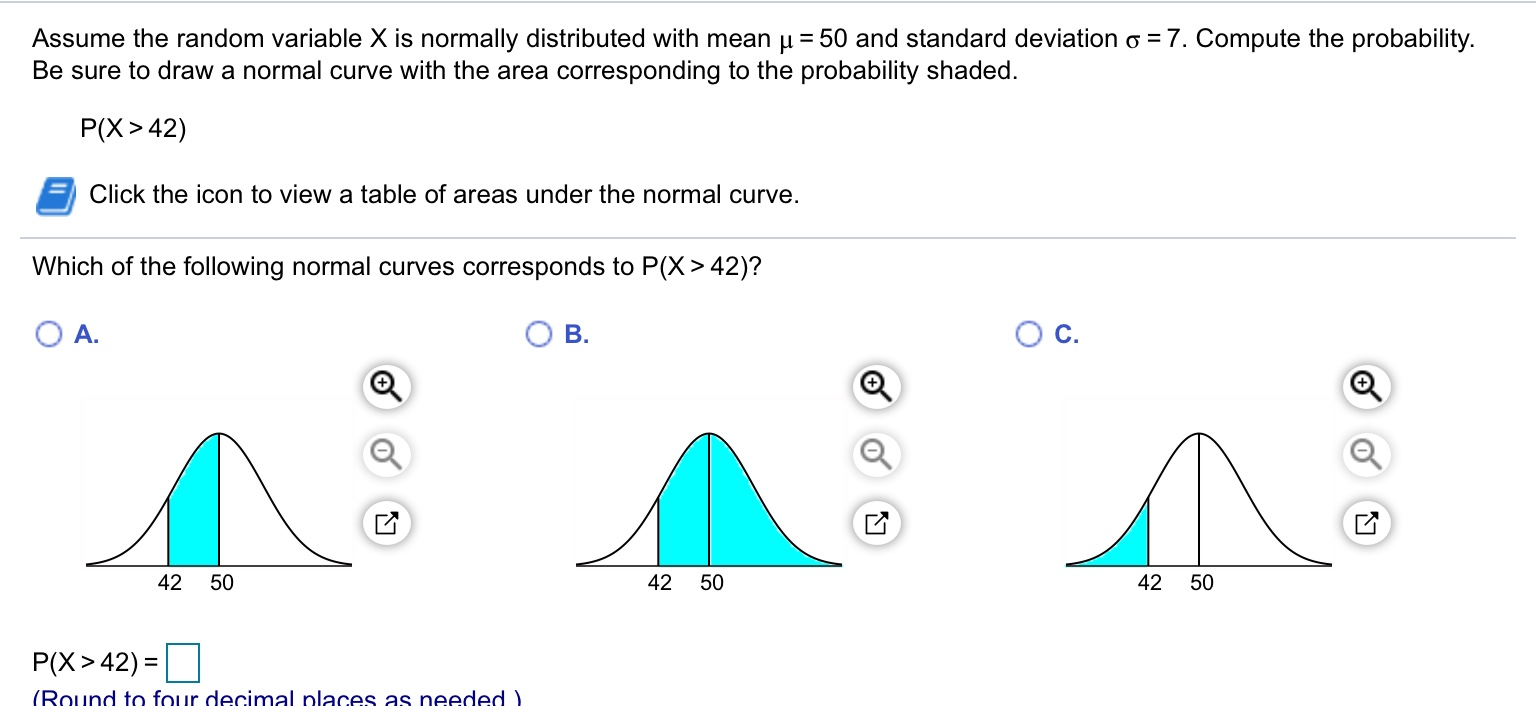# Assume the random variable X is normally distributed with mean u = 50 and standard deviation o = 7. Compute the probability.Be sure to draw a normal curve with the area corresponding to the probability shaded.P(X> 42)Click the icon to view a table of areas under the normal curve.Which of the following normal curves corresponds to P(X> 42)?Oc.A.B.42 5042 5042 50P(X>42) =(Round to four decimal places as needed )

Question
4 viewshelp_outlineImage TranscriptioncloseAssume the random variable X is normally distributed with mean u = 50 and standard deviation o = 7. Compute the probability. Be sure to draw a normal curve with the area corresponding to the probability shaded. P(X> 42) Click the icon to view a table of areas under the normal curve. Which of the following normal curves corresponds to P(X> 42)? Oc. A. B. 42 50 42 50 42 50 P(X>42) = (Round to four decimal places as needed ) fullscreen
check_circle

Step 1

Consider X be a random variable which follows normal distribution with mean, µ = 50 with sta...

### Want to see the full answer?

See Solution

#### Want to see this answer and more?

Solutions are written by subject experts who are available 24/7. Questions are typically answered within 1 hour.*

See Solution
*Response times may vary by subject and question.
Tagged in

### Statistics